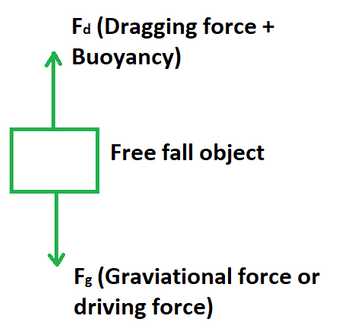Open in App
Not now

# Terminal Velocity Formula

• Last Updated : 11 May, 2022

Terminal velocity is the maximum velocity that an object can have while falling through the air. It is known that driving force is required to make the object fall under the effect of gravity. This force is susceptible to a drag force that increases with increases in velocity. As a result the object eventually achieves the terminal velocity. It occurs when the sum of dragging force and buoyancy is equal to the downward force of gravity pressing on the body.Formula

The value of terminal velocity of a free fall object varies directly with its height from the ground. It is equal to the square root of twice the product of acceleration due to gravity and height. It is denoted by the symbol vT. Its unit of measurement is m/s and dimensional formula is given by [M0L1T-1].

vT = √(2gh)

where,

vT is the terminal velocity,

g is the acceleration due to gravity,

h is the height of object.

Derivation

Suppose an object is falling from a height h with an initial velocity of zero. It is known that the final velocity is termed as terminal velocity.

We have, S = h, u = 0 and a = g.

Using the third equation of motion, we get

v2 – u2 = 2aS

v2 – 02 = 2gh

v2 = 2gh

vT = √(2gh)

This derives the formula for terminal velocity of an object.

### Sample Problems

Problem 1. Calculate the terminal velocity of an object at a height of 500 m.

Solution:

We have,

h = 500

g = 9.8

Using the formula we get,

v = √(2gh)

= √(2 × 9.8 × 500)

= √9800

= 98.99 m/s

Problem 2. Calculate the terminal velocity of an object at a height of 300 m.

Solution:

We have,

h = 300

g = 9.8

Using the formula we get,

v = √(2gh)

= √(2 × 9.8 × 300)

= √5800

= 76.88 m/s

Problem 3. Calculate the terminal velocity of an object at a height of 120 m.

Solution:

We have,

h = 120

g = 9.8

Using the formula we get,

v = √(2gh)

= √(2 × 9.8 × 120)

= √2352

= 48.49 m/s

Problem 4. Calculate the terminal velocity of an object at a height of 410 m.

Solution:

We have,

h = 410

g = 9.8

Using the formula we get,

v = √(2gh)

= √(2 × 9.8 × 410)

= √8036

= 89.64 m/s

Problem 5. Calculate the height of an object if its terminal velocity is 100 m/s.

Solution:

We have,

v = 100

g = 9.8

Using the formula we get,

v2 = 2gh

=> h = v2/2g

=> h = (100 × 100)/(2 × 9.8)

=> h = 10000/19.6

=> h = 510.2 m

Problem 6. Calculate the height of an object if its terminal velocity is 20 m/s.

Solution:

We have,

v = 20

g = 9.8

Using the formula we get,

v2 = 2gh

=> h = v2/2g

=> h = (20 × 20)/(2 × 9.8)

=> h = 400/19.6

=> h = 20.40 m

Problem 7. Calculate the height of an object if its terminal velocity is 65 m/s.

Solution:

We have,

v = 65

g = 9.8

Using the formula we get,

v2 = 2gh

=> h = v2/2g

=> h = (65 × 65)/(2 × 9.8)

=> h = 4225/19.6

=> h = 215.56 m

My Personal Notes arrow_drop_up
Related Articles